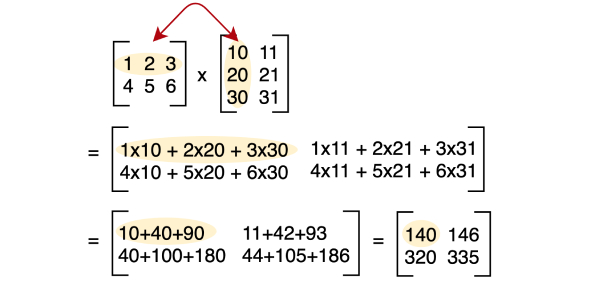Multiplication Trivia: Practice Test: Quiz!

12 Questions | Total Attempts: 99SettingsThe multiplication of whole numbers may be perceived as repeated addition; the multiplication of two numbers is equal to adding as many copies of one of them as the quantity of the other one. Both numbers are referred to as factors. One of the central properties of multiplication is the commutative property, which states the result. If you want to learn more about multiplication, take this quiz.

• 1.
8 x 10 =
• 2.
10 x 5 =
• 3.
9 x 10 =
• 4.
10 x 2 =
• 5.
10 x 10 =
• 6.
1 x 10 =
• 7.
12 x 10 =
• 8.
10 x 3 =
• 9.
10 x 7 =
• 10.
10 x 4 =
• 11.
11 x 10 =
• 12.
10 x 6 =
Related TopicsBack to top